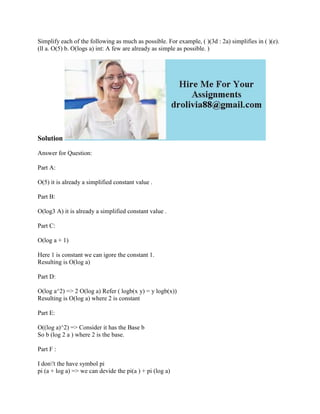Ce diaporama a bien été signalé.
Le téléchargement de votre SlideShare est en cours. ×

# Simplify each of the following as much as possible- For example- ( )(3.docx

Publicité
Publicité
Publicité
Publicité
Publicité
Publicité
Publicité
Publicité
Publicité
Publicité
PublicitéChargement dans…3
×

## Consultez-les par la suite

1 sur 2 Publicité

# Simplify each of the following as much as possible- For example- ( )(3.docx

Simplify each of the following as much as possible. For example, ( )(3d : 2a) simplifies in ( )(e). (ll a. O(5) b. O(logs a) int: A few are already as simple as possible. )
Solution
Part A:
O(5) it is already a simplified constant value .
Part B:
O(log3 A) it is already a simplified constant value .
Part C:
O(log a + 1)
Here 1 is constant we can igore the constant 1.
Resulting is O(log a)
Part D:
O(log a^2) => 2 O(log a) Refer ( logb(x y) = y logb(x))
Resulting is O(log a) where 2 is constant
Part E:
O((log a)^2) => Consider it has the Base b
So b (log 2 a ) where 2 is the base.
Part F :
I don\'t the have symbol pi
pi (a + log a) => we can devide the pi(a ) + pi (log a)
Part g:
pi(log a + 1) here 1 is constant .
pi(log a)
Part H:
pi(max(a^2, a) => pi(a^2) will be the result.
Part I:
Pi(2^n + n^100) => Pi(n^100) will be the result.
Part J:
Theta(a^2 + 2a) => a Theta(a + 2) => Theta(a^2)
Part K:
Theta( 3n^2 + 2n + log n + 1)
=> n Theta(3n + 2 + logn + 1)
Ignore constants ..
=> Theta(n^2 + logn )
Part I:
Theta(n2^n) => no modifications
Part M:
Theta(3^n + 2^n) => not sure
.

Simplify each of the following as much as possible. For example, ( )(3d : 2a) simplifies in ( )(e). (ll a. O(5) b. O(logs a) int: A few are already as simple as possible. )
Solution
Part A:
O(5) it is already a simplified constant value .
Part B:
O(log3 A) it is already a simplified constant value .
Part C:
O(log a + 1)
Here 1 is constant we can igore the constant 1.
Resulting is O(log a)
Part D:
O(log a^2) => 2 O(log a) Refer ( logb(x y) = y logb(x))
Resulting is O(log a) where 2 is constant
Part E:
O((log a)^2) => Consider it has the Base b
So b (log 2 a ) where 2 is the base.
Part F :
I don\'t the have symbol pi
pi (a + log a) => we can devide the pi(a ) + pi (log a)
Part g:
pi(log a + 1) here 1 is constant .
pi(log a)
Part H:
pi(max(a^2, a) => pi(a^2) will be the result.
Part I:
Pi(2^n + n^100) => Pi(n^100) will be the result.
Part J:
Theta(a^2 + 2a) => a Theta(a + 2) => Theta(a^2)
Part K:
Theta( 3n^2 + 2n + log n + 1)
=> n Theta(3n + 2 + logn + 1)
Ignore constants ..
=> Theta(n^2 + logn )
Part I:
Theta(n2^n) => no modifications
Part M:
Theta(3^n + 2^n) => not sure
.

Publicité
Publicité

### Simplify each of the following as much as possible- For example- ( )(3.docx

1. 1. Simplify each of the following as much as possible. For example, ( )(3d : 2a) simplifies in ( )(e). (ll a. O(5) b. O(logs a) int: A few are already as simple as possible. ) Solution Answer for Question: Part A: O(5) it is already a simplified constant value . Part B: O(log3 A) it is already a simplified constant value . Part C: O(log a + 1) Here 1 is constant we can igore the constant 1. Resulting is O(log a) Part D: O(log a^2) => 2 O(log a) Refer ( logb(x y) = y logb(x)) Resulting is O(log a) where 2 is constant Part E: O((log a)^2) => Consider it has the Base b So b (log 2 a ) where 2 is the base. Part F : I don't the have symbol pi pi (a + log a) => we can devide the pi(a ) + pi (log a)
2. 2. Part g: pi(log a + 1) here 1 is constant . pi(log a) Part H: pi(max(a^2, a) => pi(a^2) will be the result. Part I: Pi(2^n + n^100) => Pi(n^100) will be the result. Part J: Theta(a^2 + 2a) => a Theta(a + 2) => Theta(a^2) Part K: Theta( 3n^2 + 2n + log n + 1) => n Theta(3n + 2 + logn + 1) Ignore constants .. => Theta(n^2 + logn ) Part I: Theta(n2^n) => no modifications Part M: Theta(3^n + 2^n) => not sure